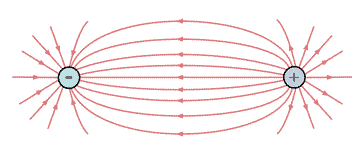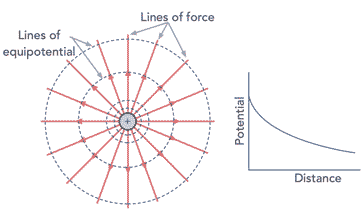# What is an Electric Field & Coulomb's Law

## Electric field, an electric property associated with each point in space when charge is present in any form.

Electric fields are everywhere and they can play a significant part in everyday life and science.

One of the most common examples seen of the existence of electric fields and their effects can be seen when a balloon is rubbed against a sweater and the balloon becomes charged and electric field is generated.

As a result of charge and the fact that like charges repel and unlike ones attract, the balloon can stick to walls. It is the electric field associated with the charge that enables the charges to repel and attract each other.

The basics of electric fields and their impact on items like electronic components and various electrical items can be quite important, so an understanding can not only be useful from having a wider view of electrical phenomena, but also from a practical viewpoints.

## What is an electric field

It is probably worth starting to look at what an electric field is by defining what it is and then explaining how it all occurs and the effects of it.

### Electric field definition:

An electric field results from each point in space where charge is present in any form. The magnitude and direction of the electric field are expressed by the value of E, called electric field strength which is often referred to as the electric field, or sometimes the electric field intensity.

The electric fields, charges and forces are explained by Coulomb's Law. As the name indicated, Coulomb's Law was developed by Charles-Augustin de Coulomb, a French Physicist. In 1785 he published three papers about electricity and magnetism and thin them he stated his law.

The development of Coulomb's Law was a major milestone in the development of the theory of electromagnetism, and one that was sued as the foundations for many more discoveries in later years.

### Coulomb's Law:

Coulomb's Law states that like charges repel and opposite charges attract with a force proportional to the product of the charges and inversely proportional to the square of the distance between them.

Despite the simplicity of the statement of Coulomb's Law, it is far reaching in its application, and it describes many aspects of electric fields: what they are; how they react and the like.

To understand Coulomb's Law and the interaction of electric charges and the resulting electric field a little more, it is possible to look at the basic concepts first and then building up from this.

It is found that an electric charge has an associated electric field and this interacts with other changes with their electric fields.

In terms fo the forces it is found that the electric force is greater if the strength of the charges is greater, i.e. charges are greater. It is also greater if the charges are closer together, and decrease if they are further away from each other. Specifically, the electric force is inversely proportional to the square of the distance between the two charges, i.e. it is an “inverse square law.”

Often the electric field lines are shown in diagrams and these indicate the electric field present at any given location.

## Coulomb's Law formula

It is often necessary to be able to express laws such as Coulomb's Law in the form of a formula or equation.

This enables a greater understanding of the law and it also enables various calculations to be made.

Where:
F = force between the two charged objects in Newtons
Q1 = charge on object 1 in coulombs
Q2 = charge on object 2 in coulombs
k = Coulomb's law constant. The value of this constant is depends on the medium in which the charged objects are immersed. For air the value is approximately 9.0 x 109 N • m2 / C2.
d = distance between two objects in metres.

It is worth noting that Coulomb's law provides an accurate description of the force between two objects that can be considered as point charges. Fortunately a charged conducting sphere acts with other charged objects as though all of its charge were located at its centre, and hence it can be considered as a point charge.

As Coulomb's law applies to point charges, the distance d in the equation above should be measured between the centres of the spheres for both objects. It is not the distance between the surfaces of the spheres. In some cases there may not bae a large difference between the two distances, but in others there will be. It is also important because the distance value is squared in the equation, and hence any discrepancies will be magnified in terms of the effect on the overall value of force.

## Electric field lines of force

An electric field is a vector quantity and can be visualised as arrows going toward or away from charges. The lines are defined as pointing radially outward, away from a positive charge, or radially inward, toward a negative charge.

The lines represent the lines of force and are often called the electric field lines. They are typically drawn as a pattern of several lines extending from the charge.Electric field lines of force between a negative and positive charge

The electric field lines point in the direction that a positive charge would accelerate if it were placed upon the line. This means that the lines are directed away from positively charged source charges and toward negatively charged source charges. To indicate these directions the electric field lines include an arrowhead that points in the appropriate direction.

In theory the pattern for an electric field would consist of an infinite number of lines. In practice, this would make any diagram unreadable, so in the interest of clarity the number of lines is usually limited. Normally just a few lines around a charge to indicate the electric field is sufficient to show the nature of the electric field in the space around the charge.

## Electric field intensity & lines of equipotential

Another important element of the electric field is the what is often called the electric field intensity. It is possible to diagrammatically represent the field intensity as equipotential lines on a diagram. These lines can be likened to the contour lines on a map. The equipotential lines are always perpendicular to the electric field.

It is also possible to represent these lines in three dimensions as elliptical spheroids of equal potential.

For a single point charge, the electric field intensity or potential gradient will be highest close to the charge, decaying as it moves further away.Electric field contours of equipotential around a point charge

In terms of moving along these lines or elliptical spheroids of equal potential, any movement requires no work as a result of movement against the electric field because this movement is always perpendicular to the electric field.

The electric field intensity can be gauged from the closeness of the lines in a similar way to the fact that close contour lines on a map indicate a very steep incline.Lightning strike represents a large discharge between positive and negative areas of chargePhoto taken from top of Petronas Towers in Kuala Lumpur Malaysia

When the electric field intensity builds up to a sufficient degree, the dielectric between the negative and positive areas of charge can even lead to a breakdown and current will flow between them giving sparks. Small sparks are relatively commonly seen around the home, but much larger ones occur in the form of lightning.

Electric fields and Coulomb's law are important in many aspects of electrical and electronics technology. Although the calculations may not be performed that often, a basic knowledge and understanding of the technology is very important. It enables the underlying reasons for some phenomena and elements of items like electronic components to be understood more fully.

More Basic Electronics Concepts & Tutorials:
Voltage     Current     Power     Resistance     Capacitance     Inductance     Transformers     Decibel, dB     Kirchoff's Laws     Q, quality factor     RF noise     Waveforms